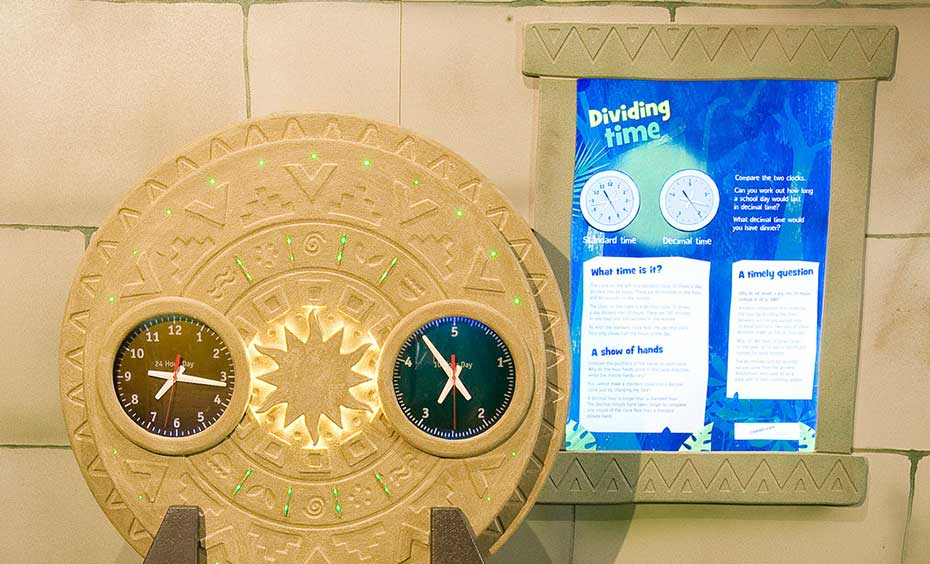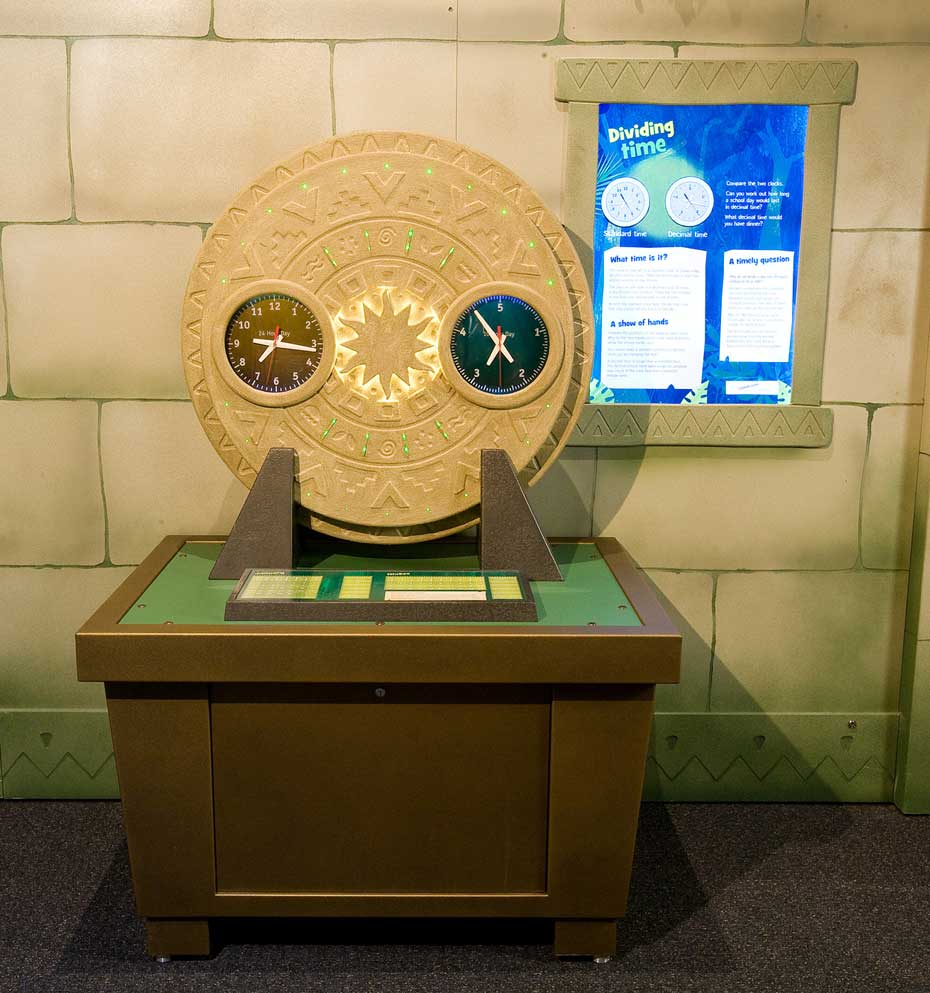# Dividing TimeStandard time clocks are based on 12 hours, but time can also be measured using a decimal clock based on 10 hours.

## How it works

Compare a standard (12 hour) clock with a decimal clock (10 hours) and try to assess the time of day using each clock.

## Things to try or ask around the exhibit

• Compare the two clocks. Can you work out how long a school day would last in decimal time?
• What decimal time would you have dinner?

## Background

A standard clock shows a day divided into 24 hours, with 60 minutes in one hour and 60 seconds in one minute.

A decimal clock shows a day divided into 10 hours. There are 100 minutes in one hour and 100 seconds in one minute. As with the standard clock face, the decimal clock face only shows half the hours in the day.

A decimal hour is longer than a standard hour. The decimal minute hand takes longer to complete one circuit of the clock face than a standard minute hand.

## Finding the science in your world

You cannot make a standard clock into a decimal clock just by changing the face!

Standard time can be shown in 12 hour cycles as AM (morning) or PM (afternoon and evening).

Alternatively, 24 hour time is used particularly by the military and medical profession to record the time of day. So 1.00 PM becomes 13:00, to represent one hour past midday (12.00).

Decimal time notation tends to be used to make labour hour for a project easier to calculate . So 30 minutes is represented as 0.50, while 1 hour and 54 minutes would be 1.9.